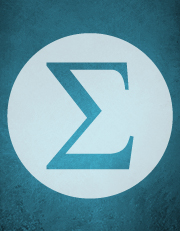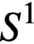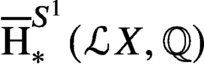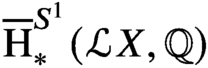Skip to main content Accessibility help
Home
Hostname: page-component-5cfd469876-wkcxx Total loading time: 0.296 Render date: 2021-06-23T18:50:56.454Z Has data issue: true Feature Flags: { "shouldUseShareProductTool": true, "shouldUseHypothesis": true, "isUnsiloEnabled": true, "metricsAbstractViews": false, "figures": true, "newCiteModal": false, "newCitedByModal": true, "newEcommerce": true }Forum of Mathematics, Sigma

# Hodge decomposition of string topology

Published online by Cambridge University Press:  13 April 2021

Corresponding
E-mail address:

## Abstract

Let X be a simply connected closed oriented manifold of rationally elliptic homotopy type. We prove that the string topology bracket on the$S^1$-equivariant homology${\overline {\text {H}}}_\ast ^{S^1}({\mathcal {L}} X,{\mathbb {Q}})$ of the free loop space of X preserves the Hodge decomposition of${\overline {\text {H}}}_\ast ^{S^1}({\mathcal {L}} X,{\mathbb {Q}})$, making it a bigraded Lie algebra. We deduce this result from a general theorem on derived Poisson structures on the universal enveloping algebras of homologically nilpotent finite-dimensional DG Lie algebras. Our theorem settles a conjecture of .

## MSC classification

Type
Algebra
Information
Creative CommonsThis is an Open Access article, distributed under the terms of the Creative Commons Attribution licence (http://creativecommons.org/licenses/by/4.0/), which permits unrestricted re-use, distribution, and reproduction in any medium, provided the original work is properly cited
Copyright
© The Author(s), 2021. Published by Cambridge University Press

## References

Avramov, L. and Halperin, S., ‘Through the looking glass: A dictionary between rational homotopy theory and local algebra’, Lecture Notes in Math. 1183 (1986), 127.CrossRefGoogle Scholar
Berest, Yu., Chen, X., Eshmatov, F. and Ramadoss, A., ‘Noncommutative Poisson structures, derived representation schemes and Calabi-Yau algebras’, Contemp. Math. 583 (2012), 219246.CrossRefGoogle Scholar
Berest, Yu., Felder, G., Patotski, S., Ramadoss, A. C. and Willwacher, T., ‘Representation homology, Lie algebra cohomology and the derived Harish-Chandra homomorphism’, J. Eur. Math. Soc. JEMS 19(9) (2017), 28112893.CrossRefGoogle Scholar
Berest, Yu., Felder, G. and Ramadoss, A., ‘Derived representation schemes and noncommutative geometry’, Contemp. Math. 607 (2014), 113162.CrossRefGoogle Scholar
Berest, Yu., Khachatryan, G. and Ramadoss, A., ‘Derived representation schemes and cyclic homology’, Adv. Math. 245 (2013), 625689.CrossRefGoogle Scholar
Berest, Yu. and Ramadoss, A., ‘Stable representation homology and Koszul duality’, J. Reine Angew. Math. 715 (2016), 143187.Google Scholar
Berest, Yu., Ramadoss, A. and Zhang, Y., ‘Dual Hodge decompositions and derived Poisson brackets’, Selecta Math. (N.S.) 23 (2017), 20292070.CrossRefGoogle Scholar
Bocklandt, R. and Le Bruyn, L., ‘Necklace Lie algebras and noncommutative symplectic geometry’, Math. Z. 240(1) (2002), 141167.CrossRefGoogle Scholar
Bökstedt, M., Hsiang, W. C. and Madsen, I., ‘The cyclotomic trace and algebraic K-theory of spaces’, Invent. Math. 111(3) (1993), 465539.CrossRefGoogle Scholar
Burghelea, D. and Fiedorowicz, Z., ‘Cyclic homology and algebraic K-theory of spaces, II’, Topology 25(3) (1986), 303317.CrossRefGoogle Scholar
Burghelea, D., Fiedorowicz, Z. and Gajda, W., ‘Adams operations in Hochschild and cyclic homology of de Rham algebra and free loop spaces’, K-Theory 4(3) (1991), 269287.CrossRefGoogle Scholar
Burghelea, D., Fiedorowicz, Z. and Gajda, W., ‘Power maps and epicyclic spaces’, J. Pure Appl. Algebra 96(1) (1994), 114.CrossRefGoogle Scholar
Calaque, D. and Rossi, C., Lectures on Duflo Isomorphisms in Lie Algebras and Complex Geometry, EMS Series of Lectures in Mathematics (European Mathematical Society, Zürich, Switzerland, 2011), viii+106.CrossRefGoogle Scholar
Chas, M. and Sullivan, D., ‘String topology’, Preprint, 1999, arXiv:math/9911159.Google Scholar
Chen, X., Eshmatov, A., Eshmatov, F. and Yang, S., ‘The derived non-commutative Poisson bracket on Koszul Calabi-Yau algebras’, J. Noncommut. Geom. 11(1) (2017), 111160.CrossRefGoogle Scholar
Crawley-Boevey, W., ‘Poisson structures on moduli spaces of representations’, J. Algebra 325 (2011), 205215.CrossRefGoogle Scholar
de Thanhoffer de Völcsey, L. and Van den Bergh, M., ‘Calabi-Yau deformations and negative cyclic homology’, J. Noncommut. Geom. 12(4) (2018), 12551291.CrossRefGoogle Scholar
Dolgushev, V. A., A Proof of Tsygan’s Formality Conjecture for an Arbitrary Smooth Manifold, Ph.D. thesis, Massachusetts Institute of Technology (2005).Google Scholar
Drinfeld, V. G., ‘On quasitriangular quasi-Hopf algebras and on a group that is closely connected with$\text{Gal}\left(\overline{\mathbb{Q}}/\mathbb{Q}\right)$’, Leningrad Math. J., 2 (1991), 829860.Google Scholar
Duflo, M., ‘Caractères des groupes et des algèbres de Lie résolubles’, Ann. Sci. Éc. Norm. Supér. (4) 3 (1970), 2374.CrossRefGoogle Scholar
Dwyer, W., Hirschhorn, P., Kan, D. and Smith, J., Homotopy Limit Functors on Model Categories and Homotopical Categories, Mathematical Surveys and Monographs vol. 113 (American Mathematical Society, Providence, RI, 2004).Google Scholar
Feigin, B. and Tsygan, B., ‘Additive K-theory and crystalline cohomology’, Funct. Anal. Appl. 19(2) (1985), 124132.CrossRefGoogle Scholar
Felix, Y., Halperin, S. and Thomas, J.-C., Rational Homotopy Theory, Graduate Texts in Mathematics vol. 205 (Springer-Verlag, New York, 2001).CrossRefGoogle Scholar
Felix, Y. and Thomas, J.-C., ‘Rational BV algebra in string topology’, Bull. Soc. Math. France 136(2) (2008), 311327.CrossRefGoogle Scholar
Felix, Y., Thomas, J.-C. and Vigué-Poirrier, M., ‘Rational string topology’, J. Eur. Math. Soc. JEMS 9 (2007), 123156.Google Scholar
Getzler, E., ‘Lie theory for nilpotent${L}_{\infty }$-algebras’, Ann. of Math. (2) 170(1) (2009), 271301.CrossRefGoogle Scholar
Getzler, E. and Kapranov, M., ‘Cyclic operads and cyclic homology’, in Geometry, Topology and Physics, Conf. Proc. Lecture Notes Geom. Topology, IV (International Press, Cambridge, MA, 1995), 167201.Google Scholar
Ginot, G., ‘On the Hochschild and Harrison (co)homology of${C}_{\infty }$-algebras and applications to string topology, in Deformation Spaces, Aspects in Mathematics, vol. E40 (Vieweg + Teubner, Wiesbaden, Germany, 2010), 151.CrossRefGoogle Scholar
Ginot, G., ‘Hodge filtration and operations in higher Hochschild (co)homology and applications to higher string topology’, in Algebraic Topology, Lecture Notes in Math. vol. 2194 (Springer, Cham, Switzerland, 2017), 1104.CrossRefGoogle Scholar
Ginzburg, V., ‘Noncommutative symplectic geometry, quiver varieties and operads’, Math. Res. Lett. 8 (2001), 377400.CrossRefGoogle Scholar
Ginzburg, V., ‘Calabi-Yau algebras’, Preprint, 2006, arXiv:math/0612139.Google Scholar
Goodwillie, T., ‘Cyclic homology, derivations, and the free loopspace’, Topology 24 (1985), 187215.CrossRefGoogle Scholar
Hamilton, A. and Lazarev, A., ‘Cohomology theories for homotopy algebras and noncommutative geometry’, Algebr. Geom. Topol. 9(3) (2009), 15031583.CrossRefGoogle Scholar
Herscovich, E., ‘Hochschild (co)homology and Koszul duality’, Preprint, 2014, arXiv:1405.2247.Google Scholar
Hirschhorn, P. S., Model categories and their localizations. Mathematical Surveys and Monographs 99, AMS, Providence, RI, 2003.Google Scholar
Jones, J.D.S., ‘Cyclic homology and equivariant homology’, Invent. Math. 87 (1987), 403423.CrossRefGoogle Scholar
Jones, J.D.S. and McCleary, J., Hochschild Homology, Cyclic Homology, and the Cobar Construction, London Math. Soc. Lecture Note Ser. vol. 175 (Cambridge University Press, Cambridge, UK, 1992), 5365.Google Scholar
Keller, B., ‘A-infinity algebras, modules and functor categories’, Contemp. Math. 406 (2006), 6793.CrossRefGoogle Scholar
Keller, B., ‘Derived invariance of higher structures on the Hochschild complex’ (2003). URL: https://webusers.imj-prg.fr/ bernhard.keller/publ/dih.pdf.Google Scholar
Kontsevich, M., Formal Noncommutative Symplectic Geometry, The Gelfand Mathematical Seminars 1990–1992 (Birkhäuser, Boston, MA, 1993), 173187.Google Scholar
Lambrechts, P. and Stanley, D., ‘Poincaré duality and commutative differential graded algebras’, Ann. Sci. Éc. Norm. Supér. (4) 41(4) (2008), 497511.CrossRefGoogle Scholar
Lefèvre-Hasegawa, K., Sur les A-infini catègories, Preprint, 2003, arxiv:math/0310337.Google Scholar
Leites, D., ‘Introduction to the theory of supermanifolds’, Uspekhi. Mat. Nauk 35(1) (1980), 1(211), 357.Google Scholar
Liao, H.-Y., Stiénon, M. and Xu, P., ‘Formality theorem for differential graded manifolds’, C. R. Math. Acad. Sci. Paris 356(1) (2018), 2743.CrossRefGoogle Scholar
Loday, J.-L., ‘Opérations sur l’homologie cyclique des algèbres commutatives’, Invent. Math. 96(1) (1989), 205230.CrossRefGoogle Scholar
Loday, J.-L., Cyclic Homology, Grundlehren Math. Wiss. vol. 301, second edn (Springer-Verlag, Berlin, 1998).CrossRefGoogle Scholar
Loday, J.-L. and Vallette, B., Algebraic Operads. Grundlehren Math. Wiss. vol. 346 (Springer, Heidelberg, 2012), xxiv+634.CrossRefGoogle Scholar
McCarthy, R., ‘On operations for Hochschild homology’, Comm. Algebra 21(8) (1993), 29472965.CrossRefGoogle Scholar
Negron, C., ‘The cup product on Hochschild cohomology via twisting cochains and applications to Koszul rings’, J. Pure Appl. Algebra 221(5) (2017), 11121133.CrossRefGoogle Scholar
Pevzner, M. and Torossian, C., ‘Isomorphisme de Duflo et cohomologie tangentielle’, J. Geom. Phys. 51(4) (2004), 486505.CrossRefGoogle Scholar
Pirashvili, T., ‘On the PROP corresponding to bialgebras’, Cah. Topol. Géom. Différ. Catég. 43(3) (2002), 221239.Google Scholar
Quillen, D., Homotopical Algebra, Lecture Notes in Math. vol. 43 (Springer-Verlag, Berlin, 1967).CrossRefGoogle Scholar
Quillen, D., ‘Rational homotopy theory’, Ann. of Math. (2) 90 (1969), 205295.CrossRefGoogle Scholar
Ramadoss, A. C., ‘The big Chern classes and the Chern character’. Internat. J. Math. 19(6) (2008), 699746.CrossRefGoogle Scholar
Ramadoss, A. C. and Zhang, Y., ‘Cyclic pairings and derived Poisson structures’, New York J. Math. 25 (2019), 144.Google Scholar
Tradler, T. and Zeinalian, M., ‘Infinity structure of Poincaré duality spaces’, Algebr. Geom. Topol. 7 (2007), 233260. With appendix by Sullivan, D..CrossRefGoogle Scholar
Tradler, T. and Zeinalian, M., ‘Algebraic string operations’, K-Theory 38(1) (2007), 5982.CrossRefGoogle Scholar
Ungheretti, M., ‘Free loop space and the cyclic bar construction’, Bull. Lond. Math. Soc. 49(1) (2017), 95101.CrossRefGoogle Scholar
Van den Bergh, M., ‘Double Poisson algebras’, Trans. Amer. Math. Soc. 360 (2008), 57115769.CrossRefGoogle Scholar
Wahl, N., ‘Universal operations in Hochschild homology’, J. Reine Angew. Math. 720 (2016), 81127.Google Scholar
Weibel, C., An Introduction to Homological Algebra, Cambridge Studies in Advanced Mathematics vol. 38 (Cambridge University Press, Cambridge, UK, 1994).CrossRefGoogle Scholar
Yekutieli, A., ‘Continuous and twisted${L}_{\infty }$ morphisms’, J. Pure Appl. Algebra 207(3) (2006), 575606.CrossRefGoogle ScholarYou have AccessOpen access

# Send article to Kindle

To send this article to your Kindle, first ensure no-reply@cambridge.org is added to your Approved Personal Document E-mail List under your Personal Document Settings on the Manage Your Content and Devices page of your Amazon account. Then enter the ‘name’ part of your Kindle email address below. Find out more about sending to your Kindle. Find out more about sending to your Kindle.

Note you can select to send to either the @free.kindle.com or @kindle.com variations. ‘@free.kindle.com’ emails are free but can only be sent to your device when it is connected to wi-fi. ‘@kindle.com’ emails can be delivered even when you are not connected to wi-fi, but note that service fees apply.

Find out more about the Kindle Personal Document Service.

Hodge decomposition of string topology
Available formats
×

# Send article to Dropbox

To send this article to your Dropbox account, please select one or more formats and confirm that you agree to abide by our usage policies. If this is the first time you use this feature, you will be asked to authorise Cambridge Core to connect with your <service> account. Find out more about sending content to Dropbox.

Hodge decomposition of string topology
Available formats
×

# Send article to Google Drive

To send this article to your Google Drive account, please select one or more formats and confirm that you agree to abide by our usage policies. If this is the first time you use this feature, you will be asked to authorise Cambridge Core to connect with your <service> account. Find out more about sending content to Google Drive.

Hodge decomposition of string topology
Available formats
×
×

#### Reply to:Submit a response

Please enter your response.

#### Your details

Please enter a valid email address.

#### Conflicting interests

Do you have any conflicting interests? *